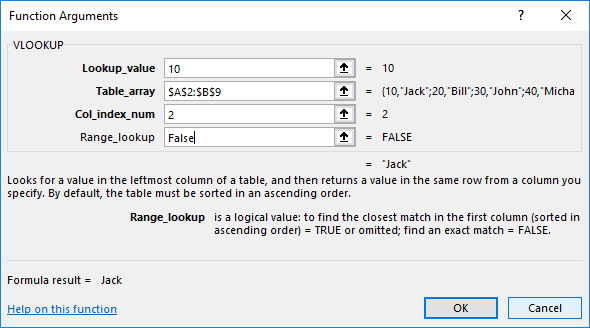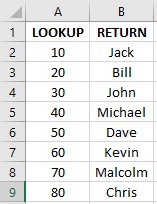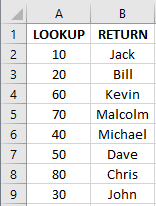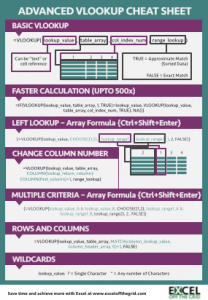# VLOOKUP: What does the True/False statement do?If you have ever needed to lookup a value from another worksheet, you will have used the VLOOKUP function.  There are other lookup functions available, but by far the most popular is VLOOKUP.  My guess is when you create this formula you enter “False” or “0” as the last argument.The True/False argument (Range_lookup argument as it is known) is optional.  You do not need to enter anything for this.  However, do not be fooled, it is only optional because if you enter nothing it will default to “True”.  In a lot of circumstances this could lead to big errors, without you even realizing it.  Therefore, I recommend you do not ever leave this argument as blank.  Always decide whether it should be “True” or “False”.

## Using False

We will look at “False” first, because it is the easier to understand.  When using “False” or “0” it will return an exact match.  Excel will start at the top of the list and work down, if the lookup value exists in the list it will return a value, but if it does not, it will return #N/A.  This seems easy to understand, if it’s there, it returns a value, if it’s not, it won’t.  Simple.

## Using True

However, what happens if we use “True” or “1” as the last statement?  We can receive all sorts of strange results.  If we do not know what VLOOKUP is doing, it can result in some significant errors in our workbooks.

Using “True” should provide an approximate match.  By approximate, it means – the next largest value that is less than the value being looked up.

Let’s look at an example:Formula Result =VLOOKUP(20,A2:B9,2,TRUE) Bill =VLOOKUP(35,A2:B9,2,TRUE) John =VLOOKUP(61,A2:B9,2,TRUE) Kevin

20 is an exact match, so Bill is returned. For the values of 35 and 61 the next largest values are 30 and 60, so the results for these values are returned.

## The Warning

Now, the time for the warning.  If you use True when your list is not sorted it may give you the wrong result.  Even if there is a exact match it can still give you the wrong result.  Lets change our example to a non-sorted list and see what happens:=VLOOKUP(20,A2:B9,2,TRUE) Bill =VLOOKUP(35,A2:B9,2,TRUE) Bill =VLOOKUP(50,A2:B9,2,TRUE) Bill =VLOOKUP(90,A2:B9,2,TRUE) John

VLOOKUP does what’s called a Binary Search.

If we are looking for 35:

• VLOOKUP with True starts in the middle at 70.  35 is less than 70 therefore, VLOOKUP goes upward and rows 6 thru 9 are eliminated.
• It moves to the middle of the top half to 20. 35 is more than 20, so row 2 is eliminated and VLOOKUP looks downward at the remaining rows.  60 is the only value left to look at.
• 35 is less than 60. So, it goes up one row to 20 for the result, which is Bill.

If we are looking for 50 (which with ‘False’ would provide an exact match), it does not return the value we might expect:

• VLOOKUP with True starts in the middle at 70.  50 is less than 70 therefore, VLOOKUP goes upward and rows 6 thru 9 are eliminated.
• It moves to the middle of the top half to 20. 50 is more than 20, so row 2 is eliminated and VLOOKUP looks downward at the remaining rows.  60 is the only value left to look at.
• 50 is less than 60. So, it goes up one row to 20 for the result, which is Bill.

If we are looking for 90:

• VLOOKUP with True starts in the middle at 70.  90 is greater than 70, so VLOOKUP moves downwards and eliminates rows 2-7.
• It moves to the middle of the bottom half to 50.  50 is less than 90, so VLOOKUP moves downwards again, and rows 6-7 are eliminated.
• It moves down to 80. 90 is greater than 80, so that row is also eliminated.
• It moves down to 30 and uses that as a result because all other options have been eliminated.

Basically, if you cannot guarantee the data you are using will always be sorted, DO NOT use “True”.  If a row could be added to the bottom of a list, which is out of order, DO NOT use “True”.

## Other lookup formulas

Other lookup formulas also have similar traits:

HLOOKUP operates exactly same as VLOOKUP, but rather than working down a list, it works it works across from left to right.

INDEX/MATCH has similar properties, but uses numbers rather than “True” or “False” and has 3 possible values

• 1 – Finds the largest value which is less than or equal to (the same as “True” for a VLOOKUP)
• 0 – Finds an exact match (the same as “False” for a VLOOKUP)
• -1 – Finds the smallest value which is greater than or equal to.  The data must be sorted in descending order.

Download the Advanced VLOOKUP Cheat Sheet.  It includes most of the tips and tricks we’ve covered in this series, including faster calculations, multiple criteria, left lookup and much more.## Other posts in the Mastering VLOOKUP Series

Don’t forget:

I’m guessing the examples in this post didn’t exactly meet your situation.  We all use Excel differently, so it’s impossible to write a post that will meet everybody’s needs.  By taking the time to understand the techniques and principles in this post (and elsewhere on this site) you should be able to adapt it to your needs.

But, if you’re still struggling you should:

1. Read other blogs, or watch YouTube videos on the same topic.  You will benefit much more by discovering your own solutions.
2. Ask the ‘Excel Ninja’ in your office.  It’s amazing what things other people know.
3. Ask a question in a forum like Mr Excel, or the Microsoft Answers Community.  Remember, the people on these forums are generally giving their time for free.  So take care to craft your question, make sure it’s clear and concise.  List all the things you’ve tried, and provide screenshots, code segments and example workbooks.
4. Use Excel Rescue, who are my consultancy partner.   They help by providing solutions to smaller Excel problems.

What next?
Don’t go yet, there is plenty more to learn on Excel Off The Grid.  Check out the latest posts:

## 4 thoughts on “VLOOKUP: What does the True/False statement do?”

1.Oz du Soleil says:

VLOOKUP/True doesn’t start at the top of the list, it starts in the middle and does what’s called a Binary Search.

In your example of looking for 35

– VLOOKUP True starts in the middle at 70.
35 is less than 70 therefore, VLOOKUP goes upward and rows 6 thru 9 are eliminated.
– It moves to the middle of the top half: 20/Bill
– 35 is more than 20. So, VLOOKUP looks at 60/Kevin
– 35 is less than 60. So, it goes back to 20/Bill for the result.

If you do a VLOOKUP/True with 90
– The search will start at 70/Malcom
– Move down to the middle of the second half since 90 is greater than 70.
– 50/Dave is less than 90.
– Move down to 80/Chris. 90 is greater than 80.
– Move down to 30/John and use that as a result because all other options have been eliminated.

One use for the binary search is in massive datasets. In a list of 1 million rows of data, it will take no more than 16 jumps to find any number.

But as you point out, the lookup array MUST be sorted.

•Excel Off The Grid says:

Hi Oz.

Yes, you are right. Good point, it has to be a binary search doesn’t it? Otherwise, there would be no speed benefit from the double VLOOKUP trick. I’ve now adopted your comments into the post.

Thank you for stopping by and commenting – I really appreciate it.

2.FrankT says:

•